# Presenting MQ sensors: low-cost gas and pollution detectors

By on February 16, 2018Let’s discover a set of low-cost gas and pollution detectors that may be easily accessed from the Arduino World.

Detecting and measuring pollution and the main gases (the ones we may come across in our daily life) is always relevant; such operations are carried out by means of specific sensors, that are often very expensive. Luckily, for a while it is possible to find low-cost, reliable sensors for sale: that’s the ones from the MQ series. They are among the most appreciated ones, since they enable – in the different applications – the detection of various kinds of gases, aerosols and particulates (such as smoke and ashes). Moreover, they are very cheap and may be easily acquired, even on the various online stores (which makes them the ones favored by those who like to experiment). Although very popular, they are however not very well documented, and it is therefore not easy to find an online library for Arduino, one that is ready and capable of correlating the signals provided with the concentrations detected in the air. For this reason, we thought to bridge this gap by proposing – in this article – an overview on the subject, and an ad hoc library for Arduino.

Let’s start by saying that MQ sensors are made of a heating element, named heater, and of an electrochemical sensor; the heater is needed in order to bring the sensor to the proper operational conditions, since only at certain temperatures the sensor’s sensitive surface (typically, a metal oxide) will react, and will let the gases and the particles (the ones we wish to detect) penetrate it.

The component reacting to the gas’ variation has been chemically treated, so to modify its electrical resistance depending on the presence of specific gases. In practice, it is a variable resistor, whose fluctuations depend on the quantity of gas found in the air in which the sensor is located.

We will later see how to correlate the variations in the sensor’s resistance to the concentration of gas, that is expressed in ppm (parts-per-million).## Features of the MQ sensors

Most sensors of the MQ series operate with working voltages that are typically at 5V, and have a lower absorption of 1 watt (5V here means less than 200 milliampere); such an absorption is mainly due to the heating element drawing power. As for some MQ sensors, the heater is powered at 2V: a low and atypical voltage, that we might obtain by using a PWM technique, starting at 5V; in practice we would drive a pulse heater (whose pulse length would be varied), along with a circuit that is based on a PWM modulator. In theory, we should be able to generate 5 V pulses with a 40% duty-cycle, by means of such a solution.

The sensors of the MQ series are mainly used in closed environments that anyway should not be particularly cold or warm, given that the heating filament’s temperature is kept quite constant by its resistance variation (it grows when the temperature grows, and vice versa) but it is not stabilized nor controlled by any circuit, therefore if the environment is too cold or warm, the obtained measurement would turn out to be altered.

Each sensor of the series reacts to more than one gas, even if usually only one or two gases excite it the most, and supply relevant resistance variations, as it is shown by the sensitivity graphs shown in these pages.

You will find a list of the main sensors, along with the gases and aerosols they detect the most, as follows:

• MQ-2 = flammable gases such as LPG and propane;
• MQ-3 =  ethanol;
• MQ-4 = methane (CH4) and natural gas;
• MQ-5 = LPG and methane;
• MQ-6 = LPG and methane;
• MQ-7 =  carbonic monoxide (CO)

and hydrogen (H2);

• MQ-8 = hydrogen (H2);
• MQ-135 = gaseous ammonia (NH3), benzene,

ethyl alcohol and carbonic dioxide (CO2).

In order to have a reliable reading, a preheating time is needed: its duration depends on the sensor. The preheat time, intended as the time that must pass before powering, is specified in the datasheet as for each sensor: it usually lasts a few hours.

The sensors of the MQ series have six pins, two of them are named A, two are named B and the last two are named H; you will see that easily in figure. The two H poles are used for powering the heater (that’s the heating element’s ends) and they must be connected to ground (GND) and to the power positive (VCC), respectively. It is advisable (but not needed) to connect the A pins together, and the same goes for the B pins; the pins are repeated in order to make the connections easier, and for the purpose of using them as a bridge on a printed circuit board.## Coupling to a microcontroller

Now, let’s see how to read the values provided by the sensors, by coupling them to a microcontroller that is supplied with an A/D converter: in order to enable the reading of the sensor’s resistance, it is needed to connect a resistor having an opportune value (the higher it is, the wider are the voltage variations, the sensor element’s resistance being equal) to the sensor element, in series; after that, let’s connect an A pin to the power and a B one to the microcontroller’s ADC input, that we will bring to ground by means of a pull-down resistor, as shown in figure With such a connection diagram, that corresponds to the creation of a voltage divider, we obtain – at the resistor’s ends – a voltage that is directly proportional to the concentration of gas and aerosol, that depends on the ratio between the sensitive element’s resistance and the one of the resistor, and on the basis of the following relationship:

Vu = VccxR/(R+Rs)

in which Vu is the voltage at the ends of the load resistor R, while Vcc is the sensor’s power voltage, and Rs is the electrical resistance of the sensitive element.In this post, we will consider the MQ-135 sensor as a reference, and we will use it in order to find the CO2 concentration in the air. In order to know our sensor better, we will test it, before connecting it to a microcontroller. Let’s power the sensor and read its electrical resistance by means of a multimeter.

Please notice that by breathing close to the sensor, its resistance will decrease; we will have to try to understand if it exists, and how to find a correlation between the variation in the resistance and the amount of gas encircling the sensor.

Unfortunately, in the datasheets of this sensors’ family we could not find any function that would solve the value for the electrical resistance read in the ppm value as for the gas we selected; but it is possible to write it by correlating the two values shown in the graph of the characteristic curve for the sensor at hand, that is found in every datasheet of the MQ series.The graph shows the value, expressed in ppm, on the x-axis; while on the y-axis it shows the ratio between the resistance of the Rs sensor and the resistance value as for a sensor, named Ro, that is exposed to 100 ppm NH3 in the air. The graph has been obtained at a 20° C temperature, with 65% humidity and a 21% oxygen (O2) concentration.

First of all, please notice that the graph is of the log-log kind; that is to say that both axes have a logarithmic scale. Since we have to search for the ppm as related to the sensor’s resistance, it will be easier to carry out a transformation of the graph, for the purpose of inverting the axes, and so to have the values for the electrical resistance on the x-axis.

In figure we propose an example showing the operation, with the inversion of the axes as for a logarithmic curve; the figure represents a series of exponential curves on a linear scale graph. Once the axes have been inverted, we have to try to understand which type of curve represents the graph. In this case, a bit of experience may help here.If we change the scale so to make it a logarithmic one, we will obtain a graph that is very similar to the one of the sensor’s sensitivity curve; more generally, it is possible to claim that the curve we are trying to obtain is an exponential function having a negative exponent. We may describe it by means of the formula (1):that as for our sensor may turn into formula (2):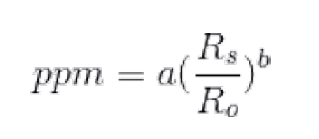What we have to do now is to find the values for the a and b coefficients, that would solve the response curve for the gas we are considering; in order to do so, we have to apply a nonlinear regression on the graph, and in particular a power regression.

First of all, we have to collect all the points in the graph; there are many tools that we may use in order to achieve this objective: a very simple tool is WebPlotDigitizer, that may be found online at the following address: https://github.com/ankitrohatgi/WebPlotDigitizer It’s free and open source, and may therefore be of great help.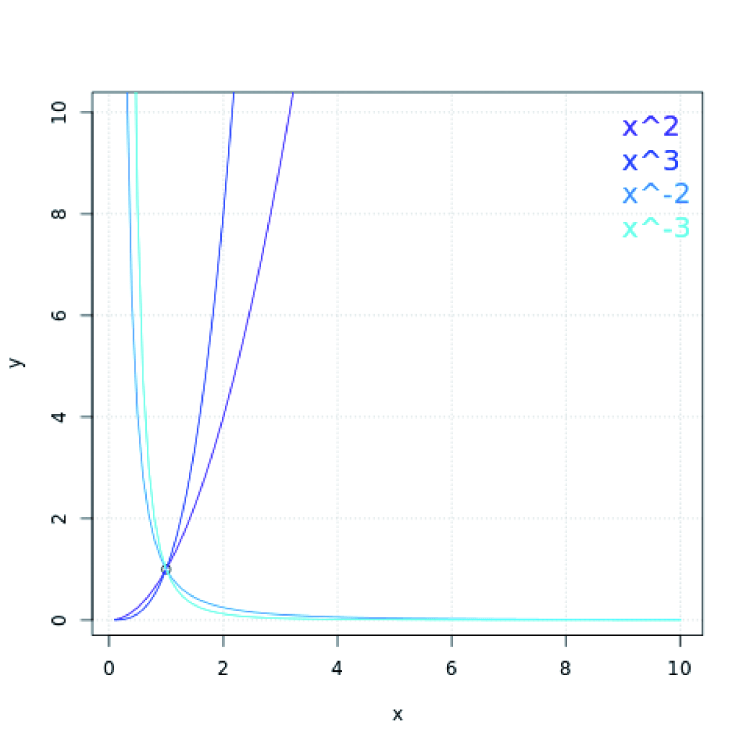We used it in order to find the value corresponding to the points in the graph. Here’s some indications on how to use it: as a first thing, we will have to extract the image from the datasheet. Let’s open the datasheet, and by means of the screen capture tool on your operating system, please save the screenshot of the characteristic curve as an image file.

We may now open the WebPlotDigitizer tool from our browser, and load the saved image.

Once the image has been loaded, we have to set the ends of the axes: the process is guided by WebPlotDigitizer’s wizard.

We now have to insert the values as for the ends of the axes we just configured, and to set the scale of the graph to “log”. As for the MQ135 sensor, the ends for the x-axis will be (10, 1000), while for the y-axis it will be (0.1, 10), as shown in figure.The next step will be the one to select the points of the graph curve we are looking for; please remember that the more points you will select, the more the function coming from the regression will be accurate. Therefore, let’s select at least ten points; finally, we will have to click on the “View Data” button and see the values for the points found.

Now that we have a list of points, we should use a tool capable of calculating the power regression. It is possible to find free online web apps that are able to do it, or we may use matlab or other applications for math calculations. We prepared a simple script for the purpose of executing these calculations, since it appeared to be the most adequate tool, as it may be easily expanded and it’s easy to use. You will find its most significant part in Listing 1.

Listing1

The script has been written in the R language, a language that is used for the statistical analysis; you may directly execute it from your browser: at the R-Fiddle’s webpage (http://www.r-fiddle.org/), you will find an R motor, capable of executing the script online. As an alternative, you may download and install R (from https://www.r-project.org/) on your development machine.Let’s copy the script in the page and set the values for the xlim, ylim and pointsdata variables, that are respectively used for the limits of the x-axis, of the y-axis and of the points of the graph.

Let’s click on the “Run Code” button, now: the script will be executed and it will be possible to read the coefficient of the curve in the console’s output, down in the page.

While on the page, it is possible to see some graphs as well, that will prove that the resolution in the regression is a valid one.

The graph in figure shows the values for the points we gathered as blue coloured, while the ones for the curve we found by executing the power regression are red coloured.Please notice that the function found doesn’t differ much from the one having the points we gathered; for such a reason we may claim that the regression we carried out respects the curve in the datasheet. Now that we found the coefficient for our correlation curve, we may look for the value of Ro: that is to say, in the case of MQ-135, the value of the sensor’s resistance when it is exposed to 100 ppm of carbon dioxide in the air.

We defined the relationship function as (2), that we show here as follows:We therefore obtain the formula (3):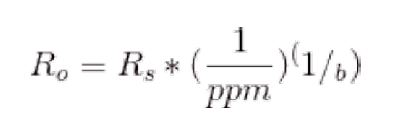The WolframAlpha search engine (please take a look at the www.wolframalpha.com website) may help us to simplify and solve these formulas.

Therefore, in order to find Ro’s value, it will be enough to read the sensor’s electrical resistance when the sensitive element is immersed in the gas we’re interested in, at the concentration we are looking for, and then to substitute the values found in the formula (3).

We chose the carbon dioxide (CO2) as a reference since it is possible to know its quantity in the air, without having to resort to expensive lab equipment. Unfortunately, the amount of open air CO2 has now slightly exceeded 400 ppm (source: https://www.co2.earth/), therefore in order to measure the sensor’s resistance when exposed to 400 ppm CO2, it will be enough to connect the sensor, to wait for the preheat time (24 hours), to leave the sensor in the open air and to later read the resistance value between the pins A and B of the sensitive element.

At this stage, a clarification is needed: as you know, we need to respect the limits of the functions in the component’s document; for example: as for the MQ-135, the CO2 gas curve is defined between 10 and 200 ppm; however, in order to be able to use a gas for which anyone may know its quantity in the air, we supposed the reference curve was valid until 1000 ppm.

In order to verify that our choice was a valid one, we then had to correlate the readings we carried out as regards the MH-14Z NDIR (Non Dispersive Infrared Sensor) sensor, having a +-5ppm resolution in the 0÷2000ppm CO2 range; you will find an excerpt in figure. The reference sensor is an infrared operating one.We would like to specify that by directly correlating the resistance value gathered from the sensor with the ones acquired from a more accurate sensor – such as the NDIR – we will obtain a lesser conversion error than the one we would obtain by using the values in the datasheet, as for the gas we are considering.

We will substitute the resistance value (expressed in ohm) found, the evaluated ppm value in the formula (3), and we will find the Ro value.

Moreover, we would like to specify that the Ro value found will be a valid one for the specific sensor whose electrical resistance we read, in fact the electrochemical sensors of this type may answer differently, depending on the batch.

Once the Ro value has been found, it will be useful to find the minimum and the maximum values of the Rs/Ro ratio, that will be useful in order to filter the correlation, with respect to the values that are out of the norm.

The minimum value as for Rs/Ro is simply obtained via the formula (4):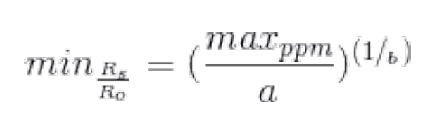On the other hand, the maximum value is obtained by solving the formula (5):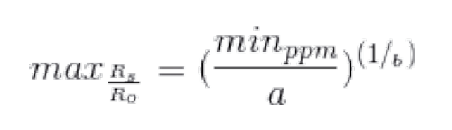We will read the maximum and minimum values for the ppm concentration in the datasheet.

The R script we supplied enables the automatic calculation of these values, simply by setting the minppm and maxppm variables.

By means of the R script we proposed, it is also possible to calculate the value for Ro and the limits of the Rs/Ro ratio.

It will be enough to input the values for the mres and mppm variables, for the sensor’s electrical resistance (expressed in ohm), and the amount of gas (expressed in ppm); the values for Ro, min Rs/Ro and max Rs/Ro will be automatically calculated and displayed in the output console.

Now that we carried out the most complex part of the work, we may bring everything inside our microcontroller and use the formulas we extracted in order to know the amount of gas found in the air.

By using the microcontroller’s ADC, that will be opportunely connected to the sensor (and as explained a few pages before), we will read the resistance value from it. Once the resistance value has been sampled, it will be enough to filter the Rs/Ro ratio according to the previously found limits, so to validate and apply the formula (2), and therefore to find the gas concentration value. In Listing 2, you will find a sketch for Arduino that may be used for this purpose; as you may see, the script code in the listing is a very simple one. As a final note, it is needed to clarify that this kind of sensor has a low accuracy and reacts to several gases found in the atmosphere. If we suppose to measure the concentration level as for the carbon monoxide, via a MQ-7 sensor, the gas level might be misrepresented because of the presence (or absence) of other gases the sensor reacts to (such as, for example, the hydrogen). However, the limited cost of the sensor makes it very useful for many projects, so that we may disregard such a detail.

Listing2

## CONCLUSIONS

In this post, we learned how to find the correlation function of the electrical resistance for the sensors of the MQ family, with the concentration value (expressed in parts-per-million) of the gas for which the sensor is most sensitive.

In particular, we examined the MQ-135 sensor’s response to the carbon dioxide, but the method we proposed may be easily used in order to find the correlation of the gases as for the other sensors of the MQ series.

## From openstore

MQ sensor